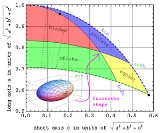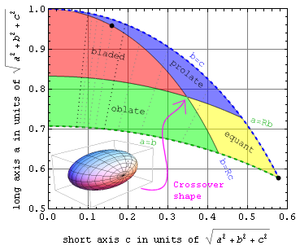xEquidimensionalEncyclopedia
Equidimensional is an adjective applied to objects that have nearly the same size or spread in multiple directions. As a mathematical concept, it may be applied to objects that extend across any number of dimensions. More specifically, it's also used to characterize the shape
Shape
The shape of an object located in some space is a geometrical description of the part of that space occupied by the object, as determined by its external boundary – abstracting from location and orientation in space, size, and other properties such as colour, content, and material...

of three-dimensional solids.

## In geologyThe word equidimensional is sometimes used by geologists to describe the shape of three-dimensional objects. In that case it is a synonym for equant. Deviations from equidimensional are used to classify the shape of convex objects like rocks or particles. For instance Th. Zingg in 1935 pointed out that if a, b and c are the long, intermediate, and short axes of a convex structure, and R is a number greater than one, then four mutually exclusive shape classes may be defined by:

Table 1: Zingg's convex object shape classes
shape category long & intermediate axes intermediate & short axes explanation example
equant b < a < R b c < b < R c all dimensions are comparable ball
prolate a > R b c < b < R c one dimension is much longer cigar
oblate b < a < R b b > R c one dimension is much shorter pancake
bladed a > R b b > R c all dimensions are very different belt

For Zingg's applications, R was set equal to . Perhaps this is an intuitively reasonable setting in general for the point at which something's dimensions become significantly unequal.

The relationship between the four categories is illustrated in the figure at right, which allows one to plot long and short axis dimensions for the convex envelope of any solid object. Perfectly equidimensional sphere
Sphere
A sphere is a perfectly round geometrical object in three-dimensional space, such as the shape of a round ball. Like a circle in two dimensions, a perfect sphere is completely symmetrical around its center, with all points on the surface lying the same distance r from the center point...

s plot in the lower right corner. Objects with equal short and intermediate axes lie on the upper bound, while objects with equal long and intermediate axes plot on the lower bound. The dotted gray and black lines correspond to integer values ranging from 2 up to 10.

The point of intersection for all four classes on this plot occurs when the object's axes a:b:c have ratios of R2:R:1, or 9:6:4 when R=. Make axis b any shorter and the object becomes prolate. Make axis b any longer and it becomes oblate. Bring a and c closer to b and the object becomes equidimensional. Separate a and c further from b and it becomes bladed.

For example, the convex envelope for some humans might plot near the black dot in the upper left of the figure.

## See also

• aspect ratio
Aspect ratio
The aspect ratio of a shape is the ratio of its longer dimension to its shorter dimension. It may be applied to two characteristic dimensions of a three-dimensional shape, such as the ratio of the longest and shortest axis, or for symmetrical objects that are described by just two measurements,...

between long and short
• equant
Equant
Equant is a mathematical concept developed by Claudius Ptolemy in the 2nd century AD to account for the observed motion of heavenly bodies....

as a noun used in astronomy
• oblate spheroid
• prolate spheroid
Prolate spheroid
A prolate spheroid is a spheroid in which the polar axis is greater than the equatorial diameter. Prolate spheroids stand in contrast to oblate spheroids...

• shape analysis
Shape analysis
This article describes shape analysis to analyze and process geometric shapes.The shape analysis described here is related to the statistical analysis of geometric shapes, to shape matching and shape recognition...

The source of this article is wikipedia, the free encyclopedia.  The text of this article is licensed under the GFDL.